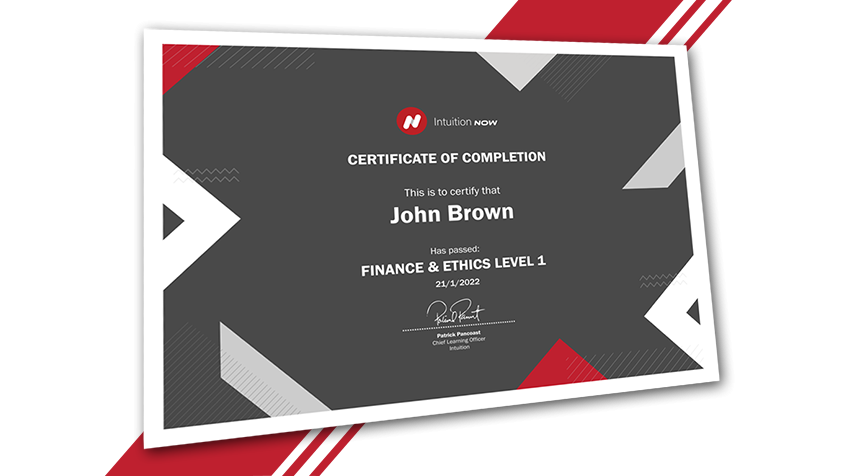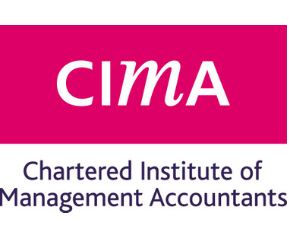# Mathematical Skills for Financial Professionals

• Level: Intermediate
• Duration: 8.4 hours
• Access: 1 Year
Learn the fundamental mathematical formulas and calculations required in banking and finance, including how to make use of Excel to perform these calculations.

• This course is only suitable for desktop use.

## Course Features

### #1 Shareable Certificate

Earn a certificate upon completion.

### #2 100% Online Learning

Start straight away and learn at your own pace.

### #3 Intermediate Level

This course is suitable for anyone with a basic understanding of mathematics.

### #4 Course Duration

Tutorial time of 8.5 hours.

### #5 Accepted for CPE

Many of the tutorials in this course are accepted for CPE by some of the world's largest financial associations.

## What You Will Learn

### 1. Indices, Exponents & Logs

• Topic 1: Indices
• Topic 2: Natural Exponential Function
• Topic 3: Logs

### 2. Logs - Excel Interactive

• Topic 1: Excel Tutorial

### 3. Mean, Median, & Mode - Excel Interactive

• Topic 1: Excel Tutorial

### 4. Probability

• Topic 1: Principles of Probability
• Topic 2: Types of Probability
• Topic 3: Expected Value of a Random Variable

### 5. Distributions

• Topic 1: Random Variables & Simple Distributions
• Topic 2: Continuous Distribution
• Topic 3: Other Continuous Probability Distributions
• Topic 4: Hypothesis Testing & Confidence Levels

### 6. Normal Distribution - Excel Interactive

• Topic 1: Excel Tutorial

### 7. Skewness & Kurtosis - Excel Interactive

• Topic 1: Excel Tutorial

### 8. Volatility Estimation

• Topic 1: Introduction to Volatility
• Topic 2: Approaches to Volatility Estimation

### 9. Volatility - Excel Interactive

• Topic 1: Excel Tutorial

### 10. Correlation Analysis

• Topic 1: Calculating Correlation
• Topic 2: Use of the Correlation Coefficient in Practice

### 11. Correlation & Covariance - Excel Interactive

• Topic 1: Excel Tutorial

### 12. Regression Analysis

• Topic 1: Uses of Regression Analysis
• Topic 2: Regression Analysis Calculations

### 13. Regression Analysis - Excel Interactive

• Topic 1: Excel Tutorial

### 14. Calculus - Differentiation

• Topic 1: Linear & Nonlinear Functions
• Topic 2: Rules of Differentiation
• Topic 3: Uses of Differentiation

### 15. Calculus - Integration

• Topic 1: Indefinite Integrals
• Topic 2: Definite Integrals
• Topic 3: Improper Integrals## Gain Industry Knowledge and a Certification

Access your learning wherever you are.
Test your knowledge throughout each tutorial with
regular review questions
End each tutorial with a short, graded test designed to enhance knowledge retention.
Gain a shareable professional certification.